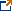# High School—Algebra and Functions

The Digital Library Instructional Learning Series links Smarter Balanced Digital Library resources with content from Interim Assessment Blocks.

The Digital Library resources on this list are intended to supplement a teacher’s core curriculum and may not address every standard assessed by the High School—Algebra and Functions Interim Assessment Block. For each resource on this list, a brief description is provided along with the Common Core State Standards (CCSS) of focus and estimated instructional time. Many of the formative assessment practices featured in these resources can be used across grades and content areas, so teachers are encouraged to explore the resources on other lists in the Digital Library Resource Collections. A Digital Library account is required to access all resources on this list. Below are the key learning goals and success criteria specifically for the resources on this list.

###### Learning Goals

Students understand how to:

• create equations that describe numbers or relationships.
• solve equations and inequalities in one variable.
• represent and solve equations and inequalities graphically.
• interpret functions that arise in applications in terms of a context.
• analyze functions using different representations.
• build a function that models a relationship between quantities.
###### Success Criteria

Students can:

• create one variable equations or inequalities arising from linear, quadratic, simple rational, and exponential functions.
• graph equations or inequalities on coordinate axes with labels and scales to represent the solution to a contextual problem.
• create equations in two or more variables to represent relationships between quantities.
• solve linear equations or inequalities in one variable with numeric coefficients.
• solve quadratic equations in one variable by taking square roots, completing the square, using the quadratic formula, or by factoring.
• recognize equivalent equations to given linear or quadratic equations in one variable.
• graph the solutions to a linear inequality in two variables as a half-plane.
• calculate and interpret the average rate of change of a function presented symbolically or as a table and estimate the rate of change of a function from a graph.
• graph functions expressed symbolically and show key features of the graph.
Title Resource Overview

Ordering SnacksCCSS of focus: A-CED.A.1-4

Estimated Instructional Time: 60 min.
This resource includes lesson plans and an activity that provide students the opportunity to work in collaborative groups to review strategies for solving problems involving multiple variables. Teams of students will be able to solve the problems using reasoning strategies and will not have to rely on writing and solving systems of equations as the only solution path. This activity serves as an introduction to solving systems of equations.

Breaking SpaghettiCCSS of focus: A-CED.A.1, F-LEA.A.2

Estimated Instructional Time: 60 min.
This resource includes lesson plans and an activity that provide students with the opportunity to work in groups to investigate the number of marbles it takes to break one or more strands of spaghetti. Students graph their results, find the line of best fit, and express this in a linear equation. Students use this equation to predict the number of marbles needed to break 100 or 500 strands of spaghetti.

Linear Functions and Nonlinearity: CBAL™ Activity Set and HandbookCCSS of focus: 7.EE.B.3, 7.EE.B.4, 8.F.A.2, 8.F.A.3, 8.F.B.4, F-IF.B.4, F-IF.B.6

Estimated Instructional Time: 300 min. per task set
This resource includes a teacher handbook and two sets of formative tasks. Both task sets elicit evidence about student ability to understand and interpret the features of linear functions. The second task set also includes early concepts of nonlinearity. All attributes of the formative assessment process are exemplified throughout each task. The teacher handbook provides detailed information on cognitive learning progressions and offers guidance based on sample responses.

The Cycle ShopCCSS of focus: A-CED.A.2, A-CED.A.3, A-REI.A.1, A-REI.B.3

Estimated Instructional Time: 540 min. (nine 60-minute lessons)
This resource includes lesson plans for two practice performance tasks, a culminating performance task, annotated student work samples, and specific supports for English language learners. The lessons support student ability to formulate and solve problems using algebra and to interpret meaning of algebraic expressions.

Optimization Problems: BoomerangsCCSS of focus: A-CED.A.2, A-CED.A.3, A-REI.C, A-REI.D

Estimated Instructional Time: 75 min.
This resource includes a lesson plan and activity for students to demonstrate their mastery creating equations that describe relationships and solve systems of inequalities. The context-based problem of maximizing income from a fundraiser is used to help students develop strategies for solving a real-world problem.

Best Buy TicketsCCSS of focus: A-CED.A, F-BF.A.1

Estimated Instructional Time: 90 min.
This resource includes lesson plans and a rubric for a performance task designed to help students apply their knowledge of creating equations and reasoning with mathematics as they determine how to choose the best printing company. Students are given time to problem solve alone, then discuss ideas in a small group, and finally groups will review and evaluate sample student responses.

Generalizing Patterns Using TilesCCSS of focus: A-CED.A, A-CED.A.1

Estimated Instructional Time: 90 min.
This resource includes a lesson plan and activity that provide students with the opportunity to determine the area of rectangular table tops by designing tile patterns using whole tiles, half tiles, and quarter tiles. Students are given time to problem solve alone, then discuss ideas in a small group, and ultimately, groups will review and evaluate sample student responses.

Dan Meyer’s “Graph Stories” for Domain, Range, and Average Rate of ChangeCCSS of focus: F.IF.A.1, F-IF.B.4, F-IF.B.5, F-IF.B.6

Estimated Instructional Time: 60 min.
This resource includes a lesson plan, presentation slides, and videos that provide students the opportunity to graph real life situations such as the length of a balloon being filled with air and the height of one’s waist off the ground when traveling down a slide. Each video or scenario is supported by a graph from which students discuss topics like domain and range of the function, intercepts, and rate of change.
Questions:   California Assessment of Student Performance and Progress Office | caaspp@cde.ca.gov | 916-445-8765
Last Reviewed: Friday, May 31, 2019
Recently Posted in Testing